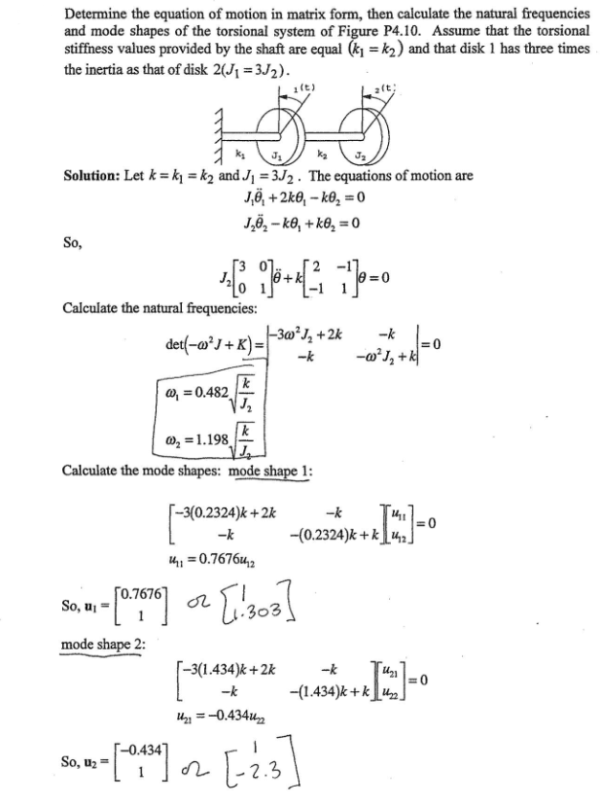Determine the equation of motion in matrix form, then calculate the natural frequencies and mode shapes of the torsional system of the Figure. Assume that the torsional stiffness values provided by the shaft are equal (k1=k2) and that disk 1 has three times the inertia as that of disk 2 (J1=3J2).Determine the equation of motion in matrix form, then calculate the natural frequencies and mode shapes of the torsional system of the Figure. Assume that the torsional stiffness values provided by the shaft are equal (k1=k2) and that disk 1 has three times the inertia as that of disk 2 (J1=3J2).

Vibrations Page 1 vibrations vibrations vibrations vibrations vibrations vibrations vibrations Vibrations Page 2